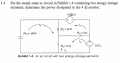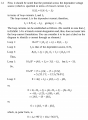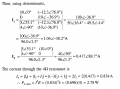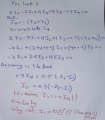# circuit analysis with dependent voltage source

#### skyline977

Joined Jan 14, 2021
29
The question is as follows:The solution is as follows:Everything is clear. I am only confused how he solved for "Loop 2:" in his solution

Below is my attempt kindly advise me of my mistake.We know that i2 does not equal 0.5*i4. Because 0.5*i4 is a VOLTAGE and not a CURRENT. so, how are they equal?
This is my confusion.
The question and answer are from: FE Exam Review: Electrical and Computer Engineering 1st Edition by Myron E. Sveum Publisher : Professional Publications, Inc. ISBN-10 : 1591260698 ISBN-13 : 978-1591260691

#### MrAl

Joined Jun 17, 2014
9,640
The question is as follows:

View attachment 229502
The solution is as follows:
View attachment 229503
View attachment 229504
Everything is clear. I am only confused how he solved for "Loop 2:" in his solution

Below is my attempt kindly advise me of my mistake.

View attachment 229505

We know that i2 does not equal 0.5*i4. Because 0.5*i4 is a VOLTAGE and not a CURRENT. so, how are they equal?
This is my confusion.
The question and answer are from: FE Exam Review: Electrical and Computer Engineering 1st Edition by Myron E. Sveum Publisher : Professional Publications, Inc. ISBN-10 : 1591260698 ISBN-13 : 978-1591260691
Hi,

I am not sure how you are reasoning about the currents I1 and I2.

First, a current though a voltage source is not always going to be equal to the same value as the source voltage itself. That's only true of a current source. So in general for a source of 5 volts the current can be -infinity to +infinity (in theory) it depends on the rest of the circuit as to what elements are drawing current and how much.
Thus, for I1, it could be anything it depends on the other elements and a complete analysis of the circuit.
For I2, it depends on the current though the dependent source MINUS the current I1.

Did you try another analysis method? For example, Nodal analysis.
I got a result that is close to what they got, i am assuming for now it is because i did not round intermediate results but kept about 16 significant figures throughout the entire analysis. I will verify (or de-verify) a little later today.

•skyline977

#### The Electrician

Joined Oct 9, 2007
2,914
The dependent source is shown using the symbol for a dependent voltage source. At the very beginning it is called a "dependent voltage source". But the rest of the solution shown treats it as a dependent current source, and the solution given is correct for a dependent current source..

•skyline977

#### MrAl

Joined Jun 17, 2014
9,640
Yes, here is a corrected drawing...

Also, notice that the current though R4 is up which effectively reverses the dependent current source polarity if we want to measure the current through R4 with polarity down instead of up.

#### Attachments

•skyline977

#### MrAl

Joined Jun 17, 2014
9,640
I just thought i would mention that if we are forced to use loop equations then i found it easier to solve for I3 and I2 in terms of I4 and that reduces the set to equations with just I1 and I4 which can then be solved for simultaneously and then we get I4 right off.

So to start and taking the sign of I4 into account::
-I4=I2+I3
so
I3=-I2-I4

and then we have simply:
I2=0.5*I4=I4/2

and then writing the equations for I1 and I3 and then substituting I1 and I3 with the above solutions we can then solve simultaneously for both I1 and I4 and that of course leaves us with I4 right away so we can calculate the power P=4*I4^2 and we are done.

The value for I4 i get is 0.833152547273 which rounded to 3 significant figures i get 0.833 so this is very slightly different than the books 0.834 probably because i did not do any intermediate rounding.
The power came out to 2.7765726681128 (or to 2.775556 which rounds to 2.78 watts if i use the rounded version 0.833) which when rounded comes out to 2.78 watts so that's the same as the book.